# List of Symbols

#### Notation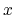Scalar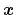Vector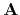Matrix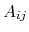Elements of the matrix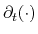Partial derivative with respect to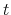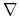Nabla operator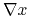Gradient of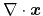Divergence of#### Physical Quantities

 Symbol Description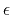Dielectric permittivity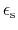Relative low frequency dielectric permittivityRelative high frequency dielectric permittivity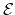Energy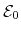Reference energy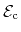Conduction band edge energy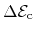Conduction band edge energy difference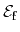Fermi energy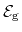Band gap energy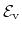Valence band edge energy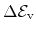Valence band edge energy difference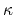Thermal conductivity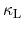Lattice contribution to thermal conductivity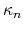Electron contribution to thermal conductivity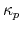Hole contribution to thermal conductivity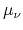Mobility of carrier type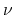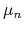Electron mobility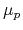Hole mobility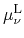mobility due to lattice scattering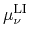mobility due to lattice and impurity scattering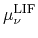mobility including lattice, impurity, and high field reduction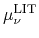mobility including lattice, impurity, and high temperature reductionNet concentration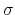Electric conductivity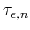Electron energy relaxation time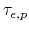Hole energy relaxation time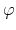Electrostatic potential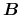Magnetic field vector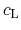Lattice heat capacity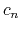Heat capacity of the electron subsystem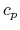Heat capacity of the hole subsystem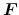Force vector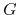Carrier generation rate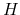Heat generation term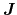Current density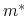Density of states effective mass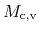Valley multiplicity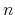Electron concentration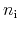Intrinsic carrier concentration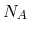Concentration of acceptors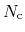Effecive density of states in the conduction band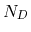Concentration of donors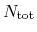Total dopant concentration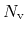Effecive density of states in the valence band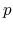Hole concentration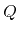Heat flowCarrier recombination rate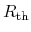Thermal resistanceTime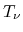Carrier temperature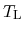Lattice temperature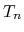Electron temperature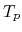Hole temperature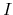Current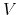Voltage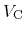Contact voltage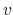Velocity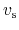Average sound velocity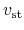Transversal sound velocity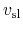Longitudinal sound velocity

#### Fundamental Constants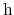Planck's constant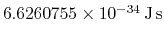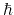Reduced Planck's constant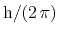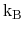Boltzmann's constant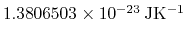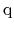Elementary charge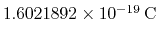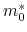Electron rest mass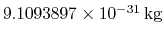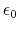Vacuum permittivityS. Vitanov: Simulation of High Electron Mobility Transistors